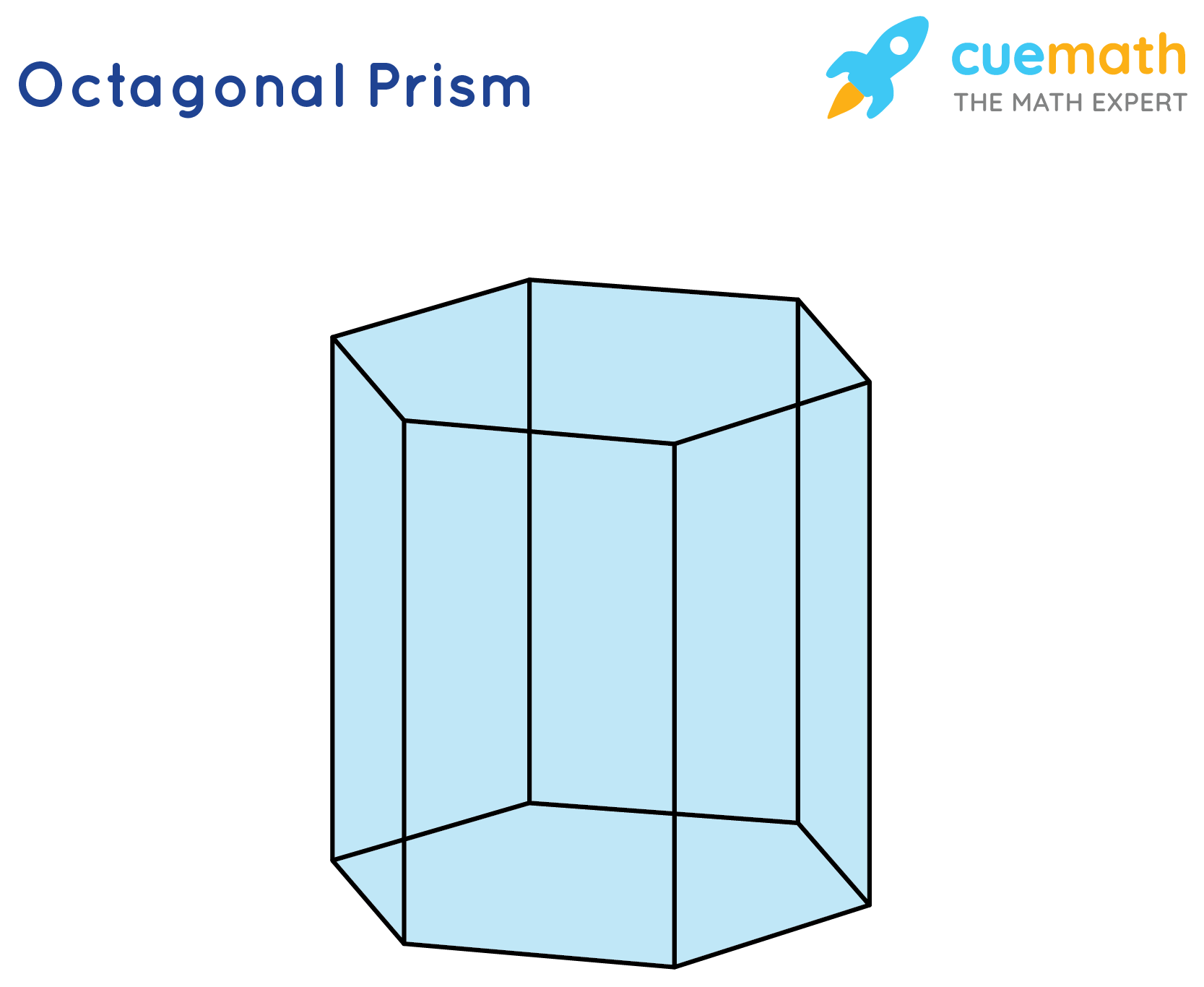# How many faces, edges, and vertices are in an octagonal prism?

## Question: How many faces, edges, and vertices are in an octagonal prism?

An octagonal prism is a three dimensional polyhedron bounded by 2 octagonal bases and 8 square sides.

## Answer: An octagonal prism has 10 faces, 24 edges and 16 vertices

Let's draw an image of octagoanal prism.

## Explanation:### Faces:

8 vertical faces + top face + bottom face = 10 faces

### Edges:

8 verticle edges + 8 top edges + 8 bottom edges = 24 edges

### Vertices:

8 vertices where the top edges meet the vertical edges + 8 vertices where the bottom edges meet the vertical edges = 16 edges